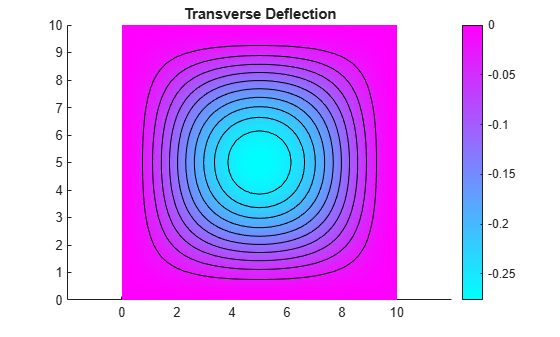Documentation

## Clamped, Square Isotropic Plate with Uniform Pressure Load

This example shows how to calculate the deflection of a structural plate acted on by a pressure loading.

### PDE and Boundary Conditions For A Thin Plate

The partial differential equation for a thin, isotropic plate with a pressure loading is

`${\nabla }^{2}\left(D{\nabla }^{2}w\right)=-p$`

where $D$ is the bending stiffness of the plate given by

`$D=\frac{E{h}^{3}}{12\left(1-{\nu }^{2}\right)}$`

and $E$ is the modulus of elasticity, $\nu$ is Poisson's ratio, and $h$ is the plate thickness. The transverse deflection of the plate is $w$ and $p$ is the pressure load.

The boundary conditions for the clamped boundaries are $w=0$ and ${w}^{\prime }=0$ where ${w}^{\prime }$ is the derivative of $w$ in a direction normal to the boundary.

The Partial Differential Equation Toolbox™ cannot directly solve the fourth order plate equation shown above but this can be converted to the following two second order partial differential equations.

`${\nabla }^{2}w=v$`

`$D{\nabla }^{2}v=-p$`

where $v$ is a new dependent variable. However, it is not obvious how to specify boundary conditions for this second order system. We cannot directly specify boundary conditions for both $w$ and ${w}^{\prime }$. Instead, we directly prescribe ${w}^{\prime }$ to be zero and use the following technique to define ${v}^{\prime }$ in such a way to insure that $w$ also equals zero on the boundary. Stiff "springs" that apply a transverse shear force to the plate edge are distributed along the boundary. The shear force along the boundary due to these springs can be written $n\cdot D\nabla v=-kw$ where $n$ is the normal to the boundary and $k$ is the stiffness of the springs. The value of $k$ must be large enough that $w$ is approximately zero at all points on the boundary but not so large that numerical errors result because the stiffness matrix is ill-conditioned. This expression is a generalized Neumann boundary condition supported by Partial Differential Equation Toolbox™

In the Partial Differential Equation Toolbox™ definition for an elliptic system, the $w$ and $v$ dependent variables are u(1) and u(2). The two second order partial differential equations can be rewritten as

`$-{\nabla }^{2}{u}_{1}+{u}_{2}=0$`

`$-D{\nabla }^{2}{u}_{2}=p$`

which is the form supported by the toolbox. The input corresponding to this formulation is shown in the sections below.

### Create the PDE Model

Create a pde model for a PDE with two dependent variables.

```numberOfPDE = 2; model = createpde(numberOfPDE);```

### Problem Parameters

```E = 1.0e6; % modulus of elasticity nu = .3; % Poisson's ratio thick = .1; % plate thickness len = 10.0; % side length for the square plate hmax = len/20; % mesh size parameter D = E*thick^3/(12*(1 - nu^2)); pres = 2; % external pressure```

### Geometry Creation

For a single square, the geometry and mesh are easily defined as shown below.

```gdm = [3 4 0 len len 0 0 0 len len]'; g = decsg(gdm,'S1',('S1')');```

Create a geometry entity.

`geometryFromEdges(model,g);`

Plot the geometry and display the edge labels for use in the boundary condition definition.

```figure; pdegplot(model,'EdgeLabels','on'); ylim([-1,11]) axis equal title 'Geometry With Edge Labels Displayed';```### Coefficient Definition

The documentation on PDE coefficients shows the required formats for the a and c matrices. The most convenient form for c in this example is ${n}_{c}=3N$ from the table where $N$ is the number of differential equations. In this example $N=2$. The $c$ tensor, in the form of an $N×N$ matrix of $2×2$ submatrices is shown below.

`$\left[\begin{array}{cccc}c\left(1\right)& c\left(2\right)& \cdot & \cdot \\ \cdot & c\left(3\right)& \cdot & \cdot \\ \cdot & \cdot & c\left(4\right)& c\left(5\right)\\ \cdot & \cdot & \cdot & c\left(6\right)\end{array}\right]$`

The six-row by one-column c matrix is defined below. The entries in the full $2×2$ a matrix and the $2×1$ f vector follow directly from the definition of the two-equation system shown above.

```c = [1 0 1 D 0 D]'; a = [0 0 1 0]'; f = [0 pres]'; specifyCoefficients(model,'m',0,'d',0,'c',c,'a',a,'f',f);```

### Boundary Conditions

`k = 1e7; % spring stiffness`

Define distributed springs on all four edges.

```bOuter = applyBoundaryCondition(model,'neumann','Edge',(1:4),... 'g',[0 0],'q',[0 0; k 0]);```

### Mesh generation

`generateMesh(model, 'Hmax', hmax);`

### Finite Element and Analytical Solutions

The solution is calculated using the `solvepde` function and the transverse deflection is plotted using the `pdeplot` function. For comparison, the transverse deflection at the plate center is also calculated using an analytical solution to this problem.

```res = solvepde(model); u = res.NodalSolution; numNodes = size(model.Mesh.Nodes,2); figure pdeplot(model,'XYData',u(1:numNodes),'Contour','on'); title 'Transverse Deflection'``````numNodes = size(model.Mesh.Nodes,2); fprintf('Transverse deflection at plate center(PDE Toolbox) = %12.4e\n',... min(u(1:numNodes,1)));```
```Transverse deflection at plate center(PDE Toolbox) = -2.7632e-01 ```

Compute analytical solution.

```wMax = -.0138*pres*len^4/(E*thick^3); fprintf('Transverse deflection at plate center(analytical) = %12.4e\n', wMax);```
```Transverse deflection at plate center(analytical) = -2.7600e-01 ```
##### SupportGet trial now